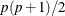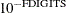#### First- or Second-Order Algorithms

The factors that go into choosing a particular optimization technique for a particular problem are complex. Trial and error can be involved.

For many optimization problems, computing the gradient takes more computer time than computing the function value. Computing the Hessian sometimes takes much more computer time and memory than computing the gradient, especially when there are many decision variables. Unfortunately, optimization techniques that do not use some kind of Hessian approximation usually require many more iterations than techniques that do use a Hessian matrix, and, as a result, the total run time of these techniques is often longer. Techniques that do not use the Hessian also tend to be less reliable. For example, they can terminate more easily at stationary points than at global optima.

Table 19.30 shows which derivatives are required for each optimization technique.

Table 19.30: Derivatives Required

Algorithm

First-Order

Second-Order

LEVMAR

x

x

TRUREG

x

x

NEWRAP

x

x

NRRIDG

x

x

QUANEW

x

-

DBLDOG

x

-

CONGRA

x

-

NMSIMP

-

-

The second-derivative methods TRUREG, NEWRAP, and NRRIDG are best for small problems where the Hessian matrix is not expensive to compute. Sometimes the NRRIDG algorithm can be faster than the TRUREG algorithm, but TRUREG can be more stable. The NRRIDG algorithm requires only one matrix withdouble words; TRUREG and NEWRAP require two such matrices. Here, p denotes the number of parameters in the optimization.

The first-derivative methods QUANEW and DBLDOG are best for medium-sized problems where the objective function and the gradient are much faster to evaluate than the Hessian. In general, the QUANEW and DBLDOG algorithms require more iterations than TRUREG, NRRIDG, and NEWRAP, but each iteration can be much faster. The QUANEW and DBLDOG algorithms require only the gradient to update an approximate Hessian, and they require slightly less memory than TRUREG or NEWRAP (essentially one matrix withdouble words).

The first-derivative method CONGRA is best for large problems where the objective function and the gradient can be computed much faster than the Hessian and where too much memory is required to store the (approximate) Hessian. In general, the CONGRA algorithm requires more iterations than QUANEW or DBLDOG, but each iteration can be much faster. Because CONGRA requires only a factor of p double-word memory, many large applications can be solved only by CONGRA.

The no-derivative method NMSIMP is best for small problems where derivatives are not continuous or are very difficult to compute.

Each optimization method uses one or more convergence criteria that determine when it has converged. An algorithm is considered to have converged when any one of the convergence criteria is satisfied. For example, under the default settings, the QUANEW algorithm will converge if ABSGCONV < 1E–5, FCONV <, or GCONV < 1E–8.